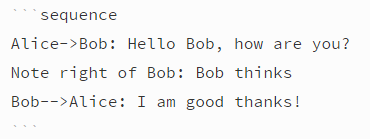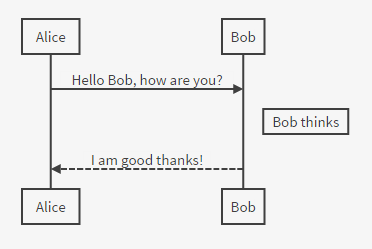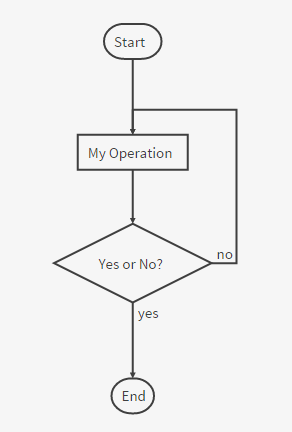# Markdown 高级语法

## 定义列表

Term 1
Term 2
:   Definition A
:   Definition B


<dl>
<dd>Term 1</dd>
<dd>Term 2</dd>
<dt>Definition A<dt>
<dt>Definition A<dt>
</dl>


## TeX公式

$\Gamma(n) = (n-1)!\quad\forall n\in\mathbb N$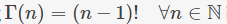TeX公式块用独占一行的两个美元符号来标记。

$$\Gamma(z) = \int_0^\infty t^{z-1}e^{-t}dt\,.$$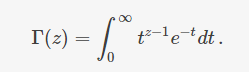<script type="text/javascript" src="https://cdn.mathjax.org/mathjax/latest/MathJax.js?config=TeX-AMS_HTML"></script>


<script type="math/tex">\Gamma(n) = (n-1)!\quad\forall n\in\mathbb N</script>

<script type="math/tex; mode=display">
\Gamma(z) = \int_0^\infty t^{z-1}e^{-t}dt\,.
</script>


TeX的语法参考请见这里

## UML图